# Nonlinear Predictive Direct Power Control Based on Space Vector Modulation of 3-Phase 3-Level Solar PV Integrated Unified Power Quality Conditioner

Nonlinear Predictive Direct Power Control Based on Space Vector Modulation of 3-Phase 3-Level Solar PV Integrated Unified Power Quality Conditioner
Applied Automation and Industrial Diagnostics Laboratory, Electrical Engineering Department, Faculty of Science and Technology, Djelfa University, Djelfa 17000, Algeria

Corresponding Author Email:
Page:
77-88
|
DOI:
https://doi.org/10.18280/ejee.240202
14 February 2022
|
Accepted:
13 April 2022
|
Published:
30 April 2022
| Citation

OPEN ACCESS

Abstract:

This paper presents a hybrid feedback linearisation-based predictive direct power control strategies of the unified power quality conditioner (UPQC) combined with a photovoltaic generator (PVG) using space vector modulation technique for power quality enhancement. The PVG-UPQC is acting as a universal conditioner for power quality enhancement and renewable energy integration simultaneously, and it mitigates harmonics in both voltage and current caused by nonlinear loads in addition to reactive power compensation. The PVG-UPQC is made up of a dc bus powered by the photovoltaic generator that connects shunt and series active power filters. The shunt filter functions as a current source and compensates for current harmonics. The series filter compensates for voltage harmonics and fluctuations such voltage sag/swell by acting as a voltage source. In order to enhance the performances of PVG-UPQC, a hybrid control method based on FL -PDPC combined with a three-level SVM controller is proposed. The aims are to deliver compensation signals faster and more accurately under a variety of load conditions, as well as eliminate voltage and current harmonics while maintaining good dynamic response. The performance of the suggested control scheme is validated by extensive simulation results obtained by Matlab/Simulink for a sensitive nonlinear load. These results are compared with those obtained with a linear PI controller proves the superiority and effectiveness of FL-PDPC controller.

Keywords:

harmonic extraction, photovoltaic generator (PVG), unified power quality conditioner (UPQC), feedback linearisation controller, space vector modulation (SVM), predictive direct power control (PDPC)

1. Introduction

Because of the increased usage of power electronic equipment in recent years, power quality has deteriorated owing to harmonic generations . At IEEE-519 and IEC-555, the standards and terminology have been thoroughly specified for power quality. The overall harmonic distortion permitted should be less than 5% according to these rules .

With the use of passive filters, the aforementioned issues can be partially overcoming [3-6]. However, this type of filter would not resolve random fluctuations in the load current and voltage waveforms. On the contrary, compensating devices, such as Static Var-Compensator (SVC), Parallel Active-Filter (PAF), Series Active-Filter (SAF) and hybrid ﬁlters are suggested to ensure power quality . However, because they can only tackle one or two power quality issues, their capabilities are typically restricted. Recent studies have demonstrated that unified power quality conditioners, which include series and shunt active filters, may tackle the majority of power quality issues at the same time .

The UPQC can keep the load end-voltage constant and prevent voltage sags/swells from entering the system . In addition, the UPQC could also effectively supply the load's reactive power requirements and suppress produced load harmonic currents, preventing them from propagating back to the utility, causing voltage and current distortion to other customers .

Different control approaches for the UPQC have been proposed. Koroglu et al.  have applied a linear control using PI controller. The control of the UPQC connected to a wind system by PI controller is suggested in Refs. [11, 12], the controller used in these papers is a conventional PI regulator, based on the kind of regulator the results demonstrated are not sufficient and the duration of the simulation can’t prove its robustness. An intelligent controller has been applied in Refs. [13, 14]. A four-wire topology of the UPQC controlled by PI has been studied by Prathyusha and Venkatesh , and da Silva et al. . In Ref.  a power quality enhancement in solar power with grid connected system using UPQC has been established. Otherwise, the system does not prove the performances of the controller under nonlinear variations in addition to the high value of the THD that reached 4.66%.

This paper has two objectives. Firstly, to develop a feedback linearisation-SVM controller for the PVG-UPQC to improve power quality. Secondly, to compare the suggested controller's performance with a PI controller to ensure the suggested controller through a varied simulation results for a nonlinear load.

The remaining part of the article is organised in the following manner: in section II, the design of different parts of the PVG-UPQC is detailed, while simulation results under different scenarios and their analysis are given in section III and the final section represents the conclusion of the present work.

2. Control Design

Figure 1 depicts the basic operation of the suggested control approach for the PVG-UPQC associated with a nonlinear load. A three-level Space Vector Modulator generates the switch control signals. The proposed controllers provide voltage references for the SVM. The instantaneous PQ theory for currents and PQ-PLL for voltages are used to establish the harmonic references [17-19]. Compensation goals include voltage and current harmonics mitigation, reactive power compensation and DC bus regulation during the bidirectional active power exchange between the PV generator, two active filters and power system grid. A detailed description of the different parts of UPQC is given hereafter.

## 1.png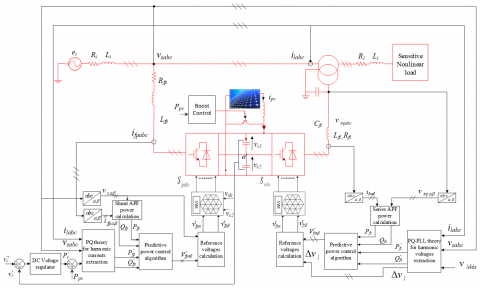Figure 1. Feedback linearization - PDPC control scheme of the PVG-UPQC

2.1 Mathematical model of GPV-UPQC

The PVG-UPQC’s dynamic model is described by a differential equation defined in α-β stationary frame. The latter is presented in Eqns. (1) and (3);

The parallel filter model is governed by the following equation.

\begin{align} & \frac{d{{i}_{fp\alpha }}}{dt}=-\frac{{{R}_{fp}}}{{{L}_{fp}}}{{i}_{fp\alpha }}-\frac{{{v}_{s\alpha }}}{{{L}_{fp}}}+\frac{{{v}_{fp\alpha }}}{{{L}_{fp}}} \\ & \frac{d{{i}_{fp\beta }}}{dt}=-\frac{{{R}_{fp}}}{{{L}_{fp}}}{{i}_{fp\beta }}-\frac{{{v}_{s\beta }}}{{{L}_{fp}}}+\frac{{{v}_{fp\beta }}}{{{L}_{fp}}} \\ \end{align}          (1)

The powers at the output of the parallel filter are given as follows:

$\left[\begin{array}{c}P_{f p} \\ Q_{f p}\end{array}\right]=\left[\begin{array}{cc}v_{f p \alpha} & v_{f p \beta} \\ v_{f p \beta} & -v_{f p \alpha}\end{array}\right]\left[\begin{array}{c}i_{f p \alpha} \\ i_{f p \beta}\end{array}\right]$              (2)

From (1) and (2) and by applying the Lie derivative method, the derivative of powers is given as:

\begin{align} & \frac{d{{P}_{fp}}}{dt}=\frac{1}{{{L}_{fp}}}\left( -{{R}_{fp}}{{P}_{fp}}+{{V}_{fp\alpha }} \right) \\ & \frac{d{{Q}_{fp}}}{dt}=\frac{1}{{{L}_{fp}}}\left( -{{R}_{fp}}{{Q}_{fp}}+{{V}_{fp\beta }} \right) \\ \end{align}          (3)

where,

\begin{align} & {{V}_{_{fp\alpha }}}={{v}_{s\alpha }}{{v}_{fp\alpha }}+{{v}_{s\beta }}{{v}_{fp\beta }}-\left( v_{s\alpha }^{2}+v_{s\beta }^{2} \right) \\ & {{V}_{fp\beta }}=-{{v}_{s\beta }}{{v}_{fp\alpha }}+{{v}_{s\alpha }}{{v}_{fp\beta }} \\ \end{align}                 (4)

It can be also written in the form (5).

${{\dot{x}}_{p}}={{f}_{p}}({{x}_{p}})+{{g}_{p}}({{x}_{p}}){{u}_{p}}$           (5)

where:

$f_{p}\left(x_{p}\right)=\left[\begin{array}{c}-\frac{R_{f p}}{L_{f p}} x_{p 1} \\ -\frac{R_{f p}}{L_{f p}} x_{p 2}\end{array}\right], g_{p}\left(x_{p}\right)=\left[\begin{array}{cc}\frac{1}{L_{f p}} & 0 \\ 0 & \frac{1}{L_{f p}}\end{array}\right],$

$x_{p}=\left[\begin{array}{c}P_{f p} \\ Q_{f p}\end{array}\right], u_{p}=\left[\begin{array}{c}V_{f p \alpha} \\ V_{f p \beta}\end{array}\right], y_{p}=\left[\begin{array}{l}y_{p 1} \\ y_{p 2}\end{array}\right]=\left[\begin{array}{l}h_{p 1} \\ h_{p 2}\end{array}\right]$

Vfpαβ, Pfp and Qfp are the voltages and active and reactive powers of the shunt filter respectively.

The DC-Bus is modeled as follows:

$\frac{dv_{dc}^{2}}{dt}=\frac{2{{P}_{c}}}{{{C}_{dc}}}$        (6)

It can be also expressed in the form of (7).

${{\dot{x}}_{dc}}={{f}_{dc}}({{x}_{dc}})+{{g}_{dc}}({{x}_{dc}}){{u}_{dc}}$              (7)

where: $f_{d c}\left(x_{d c}\right)=0, g_{d c}\left(x_{d c}\right)=\frac{2}{c_{d c}}$ and $u_{d c}\left(x_{d c}\right)=P_{c}$.

The series filter model is described by:

\begin{align} & \frac{d{{i}_{fs\alpha }}}{dt}=-\frac{{{R}_{fs}}}{{{L}_{fs}}}{{i}_{fs\alpha }}-\frac{{{v}_{inj\alpha }}}{{{L}_{fs}}}+\frac{{{v}_{fs\alpha }}}{{{L}_{fs}}} \\ & \frac{d{{i}_{fs\beta }}}{dt}=-\frac{{{R}_{fp}}}{{{L}_{fs}}}{{i}_{fs\beta }}-\frac{{{v}_{inj\beta }}}{{{L}_{fs}}}+\frac{{{v}_{fs\beta }}}{{{L}_{fs}}} \\ \end{align}             (8)

As the previous system, the derivative of powers is given as:

\begin{align} & \frac{d{{P}_{fs}}}{dt}=\frac{1}{{{L}_{fs}}}\left( -{{R}_{fs}}{{P}_{fs}}+{{V}_{fs\alpha }} \right) \\ & \frac{d{{Q}_{fs}}}{dt}=\frac{1}{{{L}_{fs}}}\left( -{{R}_{fs}}{{Q}_{fs}}+{{V}_{fs\beta }} \right) \\ \end{align}                   (9)

where,

\begin{align} & {{V}_{fs\alpha }}={{v}_{inj\alpha }}{{v}_{fs\alpha }}+{{v}_{inj\beta }}{{v}_{fs\beta }}-\left( v_{inj\alpha }^{2}+v_{inj\beta }^{2} \right) \\ & {{V}_{fs\beta }}=-{{v}_{inj\beta }}{{v}_{fs\alpha }}+{{v}_{inj\alpha }}{{v}_{fs\beta }} \\ \end{align}                     (10)

The series filter model is described by:

${{\dot{x}}_{s}}={{f}_{s}}({{x}_{s}})+{{g}_{s}}({{x}_{s}}){{u}_{s}}$               (11)

where: $f_{s}\left(x_{s}\right)=\left[\begin{array}{c}\frac{1}{C_{f s}} x_{s 1} \\ \frac{1}{C_{f s}} x_{s 2}\end{array}\right], g_{s}\left(x_{s}\right)=\left[\begin{array}{ll}\frac{1}{L_{f s}} & 0 \\ 0 & \frac{1}{L_{f s}}\end{array}\right], x_{s}=\left[\begin{array}{c}P_{f s} \\ Q_{f s}\end{array}\right], u_{s}=\left[\begin{array}{c}V_{f s \alpha} \\ V_{f s \beta}\end{array}\right] y_{s}=\left[\begin{array}{l}y_{s 1} \\ y_{s 2}\end{array}\right]=\left[\begin{array}{l}h_{s 1} \\ h_{s 2}\end{array}\right]$.

Vfsαβ, Pfs and Qfsare the voltages and active and reactive powers of the series filter respectively.

The output of the photovoltaic generator (PVG) is connected to a boost converter, as represented in Figure 2.

## 2.png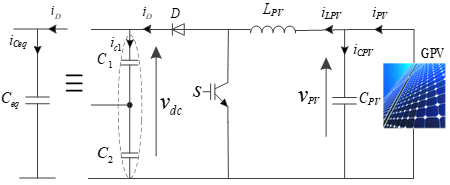Figure 2. Boost converter of the PV Generator.

The state space model for this converter is represented by the dynamic equations below:

\begin{align} & \frac{d{{v}_{pv}}}{dt}=\frac{1}{{{C}_{pv}}}{{i}_{pv}}-\frac{1}{{{C}_{pv}}}{{i}_{Lpv}} \\ & \frac{d{{i}_{Lpv}}}{dt}=\frac{1}{{{L}_{pv}}}{{v}_{pv}}-\frac{1}{{{L}_{pv}}}{{v}_{dc}} \\ \end{align}             (12)

with D is defined as duty cycle, the boost’s average model becomes as follows:

\begin{align} & \frac{d{{i}_{Lpv}}}{dt}=\frac{1}{{{L}_{pv}}}{{v}_{pv}}-\frac{1}{{{L}_{pv}}}(1-D){{v}_{dc}} \\ & \frac{d{{v}_{pv}}}{dt}=\frac{1}{{{C}_{pv}}}{{i}_{pv}}-\frac{1}{{{C}_{pv}}}(1-D){{i}_{Lpv}} \\ \end{align}                       (13)

The equations can be put in the following form:

${{\dot{x}}_{b}}={{f}_{b}}({{x}_{b}})+{{g}_{b}}({{x}_{b}}){{u}_{b}}$               (14)

where:

$f_{b}\left(x_{b}\right)=\left[\begin{array}{c}\frac{1}{L_{p v}} v_{p v} \\ \frac{1}{C_{p v}} i_{p v}\end{array}\right], g_{b}\left(x_{b}\right)=\left[\begin{array}{cc}-\frac{1}{L_{f p}} & 0 \\ 0 & -\frac{1}{C_{p v}}\end{array}\right]$,

$x_{b}=\left[\begin{array}{c}i_{L p v} \\ v_{p v}\end{array}\right], u_{b}=\left[\begin{array}{l}v_{d c} \\ i_{L p v}\end{array}\right], y_{b}=\left[\begin{array}{l}y_{b 1} \\ y_{b 2}\end{array}\right]=\left[\begin{array}{l}h_{b 1} \\ h_{b 2}\end{array}\right]$

2.2 MPPT detection algorithm

The DC-DC boost converter is commonly utilised in solar PV units, amplifying and regulating the PV panel voltage at a given level while extracting maximum power. The boost converter is controlled by a Maximum Power Point Tracking (MPPT) controller .

In this study, the P&O detection algorithm is employed because of its simplicity of use and low-cost implementation . The flowchart of P&O detection algorithm is presented in Figure 6.

The Perturb & Observe algorithm consists of changing the operating point of the PV generator by raising or reducing the duty cycle of the boost converter for the aim of measuring the output power before and after the perturbation. The algorithm perturbs the structure in the same direction as the power increases; otherwise, it perturbs the structure in the reverse direction. As shown in Figure 3.

## 3.pngFigure 3. P&O detection algorithm

There are four possible options that are presented during the tracking of the MPPT:

- if ΔP>0 and ΔV>0, V(k) is on the left of the MPP and V(k+1) will be located on a point with a higher voltage value in order to reach the MPP.

- if ΔP>0 and ΔV<0, V(k) is on the right of the MPP and V(k+1) will be located on a point with a lower voltage value in order to reach the MPP.

- if ΔP<0 and ΔV>0, V(k) is on the right of the MPP and V(k+1) will be located on a point with a lower voltage value in order to reach th MPP.

- if ΔP<0 and ΔV<0, V(k) is on the left of the MPP and V(k+1) will be located on a point with a higher voltage value in order to reach the MPP.

2.3 Harmonic identification

The identification strategy used to remove harmonics from perturbed waveforms has a big impact on the performance of the active filter [10, 19]. The methods employed for extracting harmonics are detailed in the following parts.

2.3.1 Harmonic currents identification using PQ-theory

The instantaneous power theory technique (PQ theory) is used in this study as illustrated in Figure 4.

The load's instantaneous powers are computed as follows:

$\left[\begin{array}{l}P_{l} \\ Q_{l}\end{array}\right]=\left[\begin{array}{cc}v_{s \alpha} & v_{s \beta} \\ v_{s \beta} & -v_{s \alpha}\end{array}\right]\left[\begin{array}{l}i_{l \alpha} \\ i_{l \beta}\end{array}\right]$              (15)

The powers could be written in the following way:

\left\{ \begin{align} & {{P}_{l}}={{{\bar{P}}}_{l}}+{{{\tilde{P}}}_{l}} \\ & {{Q}_{l}}={{{\bar{Q}}}_{l}}+{{{\tilde{Q}}}_{l}} \\ \end{align} \right.                (16)

For compensating reactive power and mitigating harmonics, the total reactive power ($\bar{Q}_{l}$ and $\tilde{Q}_{l}$ components) with the oscillatory component of active power ($\tilde{P}_{l}$) are chosen as compensatory power references.

## 4.png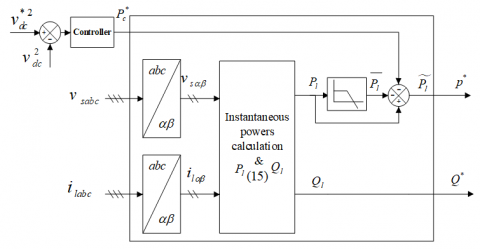Figure 4. Harmonic currents extraction scheme using PQ theory

2.3.2 Harmonic voltages identification using PQ-PLL theory

It consists of two parts in which the first is to extract voltage harmonics, and it is similar to PQ theory for currents (17):

$\left[\begin{array}{l}P_{l} \\ Q_{l}\end{array}\right]=\left[\begin{array}{cc}v_{l \alpha} & v_{l \beta} \\ v_{l \beta} & -v_{l \alpha}\end{array}\right]\left[\begin{array}{l}i_{l \alpha} \\ i_{l \beta}\end{array}\right]$          (17)

The instantaneous powers can be expressed in the following way:

\left\{ \begin{align} & P_{fs}^{*}={{{\tilde{P}}}_{l}}={{P}_{l}}-{{{\bar{P}}}_{l}} \\ & Q_{fs}^{*}={{{\tilde{Q}}}_{l}}={{Q}_{l}}-{{{\bar{Q}}}_{l}} \\ \end{align} \right.              (18)

The second part is to calculate the voltage drop across the load as follows.

$\left[\begin{array}{c}\Delta v_{l \alpha} \\ \Delta v_{l \beta}\end{array}\right]=\left[\begin{array}{l}v_{\text {des } \alpha} \\ v_{\text {des } \beta}\end{array}\right]-\left[\begin{array}{l}v_{s \alpha} \\ v_{s \beta}\end{array}\right]$            (19)

Figure 5 represents the schematic diagram of the PQ-PLL theory.

## 5.png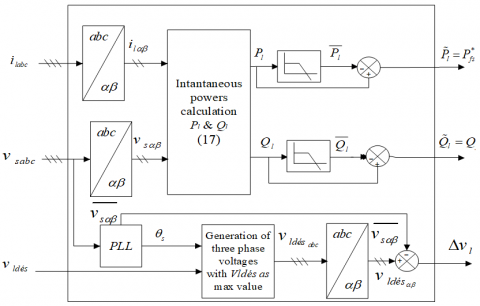Figure 5. Harmonic voltages extraction scheme using PQ-PLL theory

2.4 Feedback Linearisation Controller (FLC) synthesis

Consider the nonlinear system represented in:

\begin{align} & \dot{x}=f(x)+\sum\limits_{i=1}^{p}{{{g}_{i}}}(x){{u}_{i}}\text{, }i=1,2,....,p \\ & {{y}_{i}}={{h}_{i}}(x) \\ \end{align}              (20)

where, g(x) and f(x) are scalar functions.

The well-known method for forming the previous system's feedback linearisation law is shown in Figure 5 . The problem of determining the system's vector relative degree (13) necessitates differentiation of each output signal until one of the input signals is explicitly included in the differentiation. We define rjas the minimal integer for each output signal that has one input in $y_{j}^{\left(r_{j}\right)}$ :

$y_{j}^{({{r}_{j}})}={{L}_{f}}^{{{r}_{j}}}h(x)+\sum\limits_{i=1}^{p}{{{L}_{gi}}({{L}_{f}}^{{{r}_{j}}-1}{{h}_{j}}(x))}{{u}_{i}}\,\,\,j=1,2,.....,p\,$            (21)

where: Lfhj and $L_{g i}\left(L_{f}{ }^{r_{j-1}-1} h_{j}\right)$ represent the Lie derivatives of h with regard to f and g.

The global relative degree (r) is equal to the total of all relative degrees calculated using (20). It should be less than or equal to the system's order: $r=\sum_{j=1}^{p} r_{j} \leq n$.

The formula (20) can be represented in its matrix form to obtain the expression of linearising law u that permits making the relationship between inputs and outputs linear:

${{[y_{1}^{{{r}_{j}}}\text{ }...\text{ }...\text{ }y_{p}^{{{r}_{p}}}]}^{t}}=\zeta (x)+D(x)u\text{ }$             (22)

where:

\zeta (x)=\left( \begin{align} & {{L}_{f}}^{{{r}_{1}}}{{h}_{1}}(x) \\ & {{L}_{f}}^{{{r}_{2}}}{{h}_{2}}(x) \\ & \text{ }. \\ & \text{ }. \\ & \text{ }. \\ & {{L}_{f}}^{{{r}_{p}}}{{h}_{p}}(x) \\ \end{align} \right)

And

D(x)=\left( \begin{align} & {{L}_{{{g}_{1}}}}{{L}_{f}}^{{{r}_{1}}-1}{{h}_{1}}\,\,\,\,\,\,\,{{L}_{{{g}_{2}}}}{{L}_{f}}^{{{r}_{1}}-1}{{h}_{1}}\,\,\,\,\,\,\,.\,\,\,\,\,\,\,.\,\,\,\,\,\,\,.\,\,\,\,\,\,\,.\,\,\,\,\,\,\,{{L}_{{{g}_{p}}}}{{L}_{f}}^{{{r}_{1}}-1}{{h}_{1}}\, \\ & {{L}_{{{g}_{1}}}}{{L}_{f}}^{{{r}_{2}}-1}{{h}_{2}}\,\,\,\,\,\,{{L}_{{{g}_{2}}}}{{L}_{f}}^{{{r}_{2}}-1}{{h}_{2}}\,\,\,\,\,\,.\,\,\,\,\,\,\,.\,\,\,\,\,\,\,.\,\,\,\,\,\,\,.\,\,\,\,\,\,{{L}_{{{g}_{p}}}}{{L}_{f}}^{{{r}_{2}}-1}{{h}_{2}}\, \\ & \,\,\,\,\,\,\,\,\,\,\,.\,\,\,\,\,\,\,\,\,\,\,\,\,\,\,\,\,\,\,\,\,\,\,\,\,\,\,\,\,.\,\,\,\,\,\,\,\,\,\,\,\,\,\,\,\,\,.\,\,\,\,\,\,\,.\,\,\,\,\,\,\,.\,\,\,\,\,\,\,.\,\,\,\,\,\,\,\,\,\,\,\,\,\,\,\,\,\,\,.\,\,\,\,\,\,\,\,\,\, \\ & {{L}_{{{g}_{1}}}}{{L}_{f}}^{{{r}_{p}}-1}{{h}_{p}}\,\,\,\,{{L}_{{{g}_{2}}}}{{L}_{f}}^{{{r}_{p}}-1}{{h}_{p}}\,\,\,\,\,.\,\,\,\,\,\,\,.\,\,\,\,\,\,\,.\,\,\,\,\,\,\,.\,\,\,\,\,\,{{L}_{{{g}_{p}}}}{{L}_{f}}^{{{r}_{p}}-1}{{h}_{p}}\, \\ \end{align} \right)

D(x) is called the decoupling matrix system.

The linearizing control law has the following form :

$u=D{{(x)}^{-1}}(-\zeta (x)+v)\text{ }$          (23)

It is important to note that linearisation is only possible if the decoupling matrix D(x) is reversible. Figure 6 shows the linearised system's block diagram.

## 6.png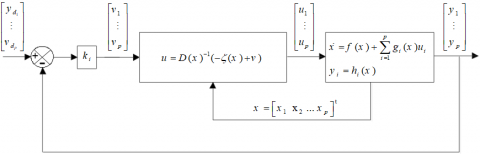Figure 6. Block diagram of linearised MIMO system

2.4.1 Boost FLC synthesis

Each output derivative is given by:

${{\dot{y}}_{j}}={{L}_{f}}^{{}}h(x)+\sum\limits_{i=1}^{2}{{{L}_{gi}}({{L}_{f}}^{{}}{{h}_{j}}(x))}{{u}_{i}}\,\,\,j=1,2\,$          (24)

Then, the matrix form of (24) can be expressed as:

$\left[\begin{array}{c}\dot{y}_{b 1} \\ \dot{y}_{b 2}\end{array}\right]=\left[\begin{array}{c}\frac{1}{L_{p v}} x_{b 1} \\ \frac{1}{C_{p v}} x_{b 2}\end{array}\right]+\left[\begin{array}{cc}-\frac{1}{L_{p v}} & 0 \\ 0 & -\frac{1}{C_{p v}}\end{array}\right]\left[\begin{array}{l}u_{b 1} \\ u_{b 2}\end{array}\right]$                     (25)

The decoupling matrix is reversible (det(Db(x)) ≠0), then the linearizing control law can be given as:

$u_{b}=\left[\begin{array}{l}u_{b 1} \\ u_{b 2}\end{array}\right]=D_{b}\left(x_{b}\right)^{-1}\left[-\zeta_{b}\left(x_{b}\right)+\left[\begin{array}{l}v_{b 1} \\ v_{b 2}\end{array}\right]\right]$            (26)

By applicating the linearisation law, we obtain the following decoupled linear system:

$\left[\begin{array}{l}\dot{y}_{b 1} \\ \dot{y}_{b 2}\end{array}\right]=\left[\begin{array}{l}\boldsymbol{v}_{b 1} \\ \mathcal{v}_{b 2}\end{array}\right]$               (27)

The control law utilised for tracking the reference is:

\begin{align} & {{v}_{b1}}={{k}_{b1}}(i_{Lpv}^{*}-{{i}_{Lpv}})+\frac{di_{Lpv}^{*}}{dt} \\ & {{v}_{b2}}={{k}_{b2}}(v_{pv}^{*}-{{v}_{pv}})+\frac{dv_{pv}^{*}}{dt} \\ \end{align}               (28)

where, kb1 and kb2 are positive constants.

From (27) and (28), the control law is given by:

\begin{align} & v_{dc}^{*}={{v}_{pv}}+{{L}_{pv}}({{k}_{b1}}(i_{Lpv}^{*}-{{i}_{Lpv}})+\frac{di_{Lpv}^{*}}{dt}) \\ & i_{Lpv}^{*}={{i}_{pv}}+{{C}_{pv}}({{k}_{b2}}(v_{pv}^{*}-{{v}_{pv}})+\frac{dv_{pv}^{*}}{dt}) \\ \end{align}                         (29)

2.4.2 DC voltage FLC synthesis

The design of the DC link voltage controller is based on (5). The derivative of the output y=h = vdc2 is computed as:

$\overset{\centerdot }{\mathop{y}}\,={{L}_{f}}h(x)+{{L}_{g}}h(x)u=\frac{2}{{{C}_{dc}}}P_{dc}^{{}}$                 (30)

The control Pdc is figured in (30); hence, its relative degree r =1. The relative degree of this output is equal to the order of the system which clearly corresponds to an exact linearisation .

The control is then determined by:

$P_{dc}^{*}=\frac{{{C}_{dc}}}{2}v$               (31)

where: $\dot{y}=v$

For the problem of trajectory tracking defined by vdc*(t), the linearizing control law v is defined by:

$v={{k}_{dc}}(v_{dc}^{{{*}^{2}}}-v_{dc}^{2})+\frac{dv_{dc}^{*2}}{dt}$                (32)

where, kdc is a positive constant.

2.5 Predictive Direct Power Control (PDPC)

2.5.1 Parallel Powers PDPC synthesis

The suggested predictive DPC technique is based on a predictive control algorithm that makes instantaneous active and reactive powers equal to their reference values at each sampling period by computing the PVG-UPQC average voltage vector. As a result, as illustrated in Figure 7, instantaneous active and reactive power measurements and commands are employed as input data variables for the predictive control algorithm. At the beginning of each sampling period Te, the PVG-UPQC average voltage vector $V_{f p \alpha \beta}$, which allows cancellation of instantaneous active and reactive power tracking errors at the end of the sampling period, is computed. Then, 3-level SVM technique is used to generate a sequence of switching states to achieve the control objective with constant switching frequency.

PDPC requires a predictive model of the instantaneous power behavior, which is described in the following steps.

If the sampling period Te is infinitely small compared with the fundamental period. The discretization of the Eqns. (3) and (9) yields:

\begin{align} & {{P}_{fp}}(k+1)-{{P}_{fp}}(k)=\frac{{{T}_{e}}}{{{L}_{fp}}}(-{{R}_{fp}}{{P}_{fp}}(k)+{{V}_{fp\alpha }}(k)) \\ & {{Q}_{fp}}(k+1)-{{Q}_{fp}}(k)=\frac{{{T}_{e}}}{{{L}_{fp}}}(-{{R}_{fp}}{{Q}_{fp}}(k)+{{V}_{fp\beta }}(k)) \\ \end{align}               (33)

Since the control objective is to force active and reactive powers to be equal to their reference values at the next sampling period, Eq. (33) can be rewritten as follows:

\begin{align} & P_{fp}^{*}(k+1)={{P}_{fp}}(k+1)=\frac{{{T}_{e}}}{{{L}_{fp}}}(-{{R}_{fp}}{{P}_{fp}}(k)+{{V}_{fp\alpha }}(k))+{{P}_{fp}}(k) \\ & Q_{fp}^{*}(k+1)={{Q}_{fp}}(k+1)=\frac{{{T}_{e}}}{{{L}_{fp}}}(-{{R}_{fp}}{{Q}_{fp}}(k)+{{V}_{fp\beta }}(k)) \\ & +{{Q}_{fp}}(k) \\ \end{align}                   (34)

Using (34), the required GPV-UPQC average voltage is given by:

\begin{align} & {{V}_{fp\alpha }}(k)={{R}_{fp}}{{P}_{fp}}(k)+\frac{{{L}_{fp}}}{{{T}_{e}}}(P_{fp}^{*}(k+1)-{{P}_{fp}}(k)) \\ & {{V}_{fp\beta }}(k)={{R}_{fp}}{{Q}_{fp}}(k)+\frac{{{L}_{fp}}}{{{T}_{e}}}(Q_{fp}^{*}(k+1)-{{Q}_{fp}}(k)) \\ \end{align}                        (35)

By using a linear extrapolation, the instantaneous power references at the next sampling period (k+1) can be estimated as shown in Figure 7.

## 7.png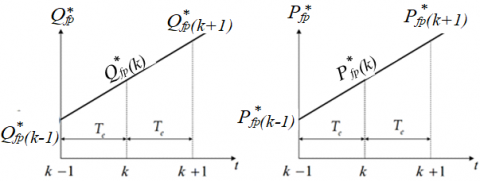Figure 7. Predictive value estimation of reference powers

The references for estimated power are as follows:

\begin{align} & P_{fp}^{*}(k+1)=2P_{fp}^{*}(k)-P_{fp}^{*}(k-1) \\ & Q_{fp}^{*}(k+1)=2Q_{fp}^{*}(k)-Q_{fp}^{*}(k-1) \\ \end{align}                (36)

The discrete PDPC control law which provides the required PVG-UPQC average voltage vector to be applied during each sampling period is given by the following equation:

\begin{align} & V_{fp\alpha }^{*}(k)={{R}_{fp}}{{P}_{fp}}(k)+\frac{{{L}_{fp}}}{{{T}_{e}}}(\Delta P_{fp}^{*}(k)+{{e}_{{{P}_{fp}}}}(k)) \\ & V_{fp\beta }^{*}(k)={{R}_{fp}}{{Q}_{fp}}(k)+\frac{{{L}_{fp}}}{{{T}_{e}}}(\Delta Q_{fp}^{*}(k)+{{e}_{{{Q}_{fp}}}}(k)) \\ \end{align}                  (37)

where, $e_{P_{f p}}(k)$ et $e_{Q_{f p}}(k)$ are the actual active and reactive power tracking errors defined as:

\begin{align} & {{e}_{{{P}_{fp}}}}(k)=P_{fp}^{*}(k)-{{P}_{fp}}(k) \\ & {{e}_{{{Q}_{fp}}}}(k)=Q_{fp}^{*}(k)-{{Q}_{fp}}(k) \\ \end{align}                     (38)

$\Delta P_{f p}^{*}(k)$ et $\Delta Q_{f p}^{*}(k)$ are the actual change in active and reactive power references given by:

\begin{align} & \Delta P_{fp}^{*}(k)=P_{fp}^{*}(k)-P_{fp}^{*}(k-1) \\ & \Delta Q_{fp}^{*}(k)=Q_{fp}^{*}(k)-Q_{fp}^{*}(k-1) \\ \end{align}                 (39)

Once the intermediate voltages $V_{f p \alpha}^{*}$ and $V_{f p \beta}^{*}$ are obtained, the reference voltages $v_{f p \alpha}^{*}$ and $v_{f p \beta}^{*}$ can be calculated using (4) as follows:

$v_{fp\alpha }^{*}=\frac{{{v}_{s\alpha }}}{v_{s\alpha }^{2}+v_{s\beta }^{2}}V_{fp\alpha }^{*}-\frac{{{v}_{s\beta }}}{v_{s\alpha }^{2}+v_{s\beta }^{2}}V_{fp\beta }^{*}+{{v}_{s\alpha }}$                    (40)

and,

$v_{fp\beta }^{*}=\frac{{{v}_{s\beta }}}{v_{s\alpha }^{2}+v_{s\beta }^{2}}V_{fp\alpha }^{*}+\frac{{{v}_{s\alpha }}}{v_{s\alpha }^{2}+v_{s\beta }^{2}}V_{fp\beta }^{*}+{{v}_{s\beta }}$                 (41)

2.5.2 Series powers PDPC synthesis

By the same manner, the control law used for tracking is given by:

\begin{align} & V_{fs\alpha }^{*}(k)={{R}_{fs}}{{P}_{fs}}(k)+\frac{{{L}_{fs}}}{{{T}_{e}}}(\Delta P_{fs}^{*}(k)+{{e}_{{{P}_{fs}}}}(k)) \\ & V_{fs\beta }^{*}(k)={{R}_{fs}}{{Q}_{fs}}(k)+\frac{{{L}_{fs}}}{{{T}_{e}}}(\Delta Q_{fs}^{*}(k)+{{e}_{{{Q}_{fs}}}}(k)) \\ \end{align}                       (42)

$v_{fs\alpha }^{*}=\frac{{{v}_{inj\alpha }}}{v_{inj\alpha }^{2}+v_{inj\beta }^{2}}V_{fs\alpha }^{*}-\frac{{{v}_{inj\beta }}}{v_{inj\alpha }^{2}+v_{inj\beta }^{2}}V_{fs\beta }^{*}+{{v}_{inj\alpha }}$                       (43)

and,

$v_{fs\beta }^{*}=\frac{{{v}_{inj\beta }}}{v_{inj\alpha }^{2}+v_{inj\beta }^{2}}V_{fs\alpha }^{*}+\frac{{{v}_{inj\alpha }}}{v_{inj\alpha }^{2}+v_{inj\beta }^{2}}V_{fs\beta }^{*}+{{v}_{inj\beta }}$                 (44)

2.6 Three-level space vector modulation

In this part, the space vector modulation (SVM) algorithm is described to produce the switches PWM control signals (sa, sb and sc). represents the space vector stated for a three-level inverter in α-β frame. There exist 27 states: 12 on the outer hexagon, 12 on the inner hexagon and 3 zero-states in the center. For these states, ‘2’, ‘0’ and ‘1’ imply that the output voltage can take ‘ vdc/2’, ‘0’ and ‘- vdc /2’, respectively.

## 8.png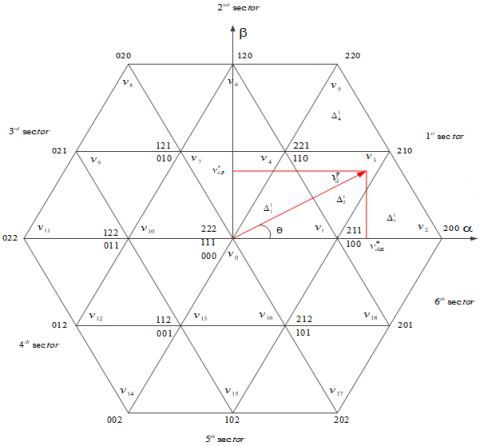Figure 8. Three-level space-vector diagram

As illustrated in Figure 8, each sector is made up of four triangular areas. The goal behind space vector modulation is to reconstruct the reference voltage vector from its three nearby vectors in such a way that the sum of these various vectors equals the reference voltage vector. As a result, the initial step must be to locate the reference voltage vector. This procedure may be broken down into two steps where the first identifies the sector number, and then it calculates the triangle in which the vector is placed [20-22].

## 9.pngFigure 9. Vector diagram in the first sector

As illustrated in Figure 9, the magnitude and angle of the reference voltage vector are determined as:

$\mathcal{V}_{d}^{*}=\sqrt{\mathcal{V}_{d\alpha }^{*2}+v_{d\beta }^{*2}}$          (45)

$\theta =\tan {{2}^{-1}}\left( \frac{v_{d\beta }^{*}}{v_{d\alpha }^{*}} \right)$            (46)

1. First Step: Sector Determination

The sector numbers are given in the following equation:

$S=ceil(\frac{\theta }{\pi /3})\in \{1,2,3,4,5,6\}$                (47)

where ceil is a function that rounds a number to the next larger integer.

1.  Second Step: Triangle Identification

The reference vector is projected on the two axes doing $\pi / 3 \operatorname{rad}$ between them. The projected components are normalized by Eqns. (48) and (49) as follows:

$v_{1}^{*S}=2\frac{v_{d}^{*}}{\sqrt{2/3}{{v}_{dc}}}\left( \cos (\theta -(S-1)\frac{\pi }{3})-\frac{1}{\sqrt{3}}\sin (\theta -(S-1)\frac{\pi }{3}) \right)$              (48)

$v_{2}^{*S}=2\frac{v_{d}^{*}}{\sqrt{2/3}{{v}_{dc}}}\left( \frac{2}{\sqrt{3}}\sin (\theta -(S-1)\frac{\pi }{3}) \right)$                 (49)

In order to determine the number of the triangle in a sector S, the following two integers should be defined as [23, 24]:

\begin{align} & l_{1}^{S}=\operatorname{int}\left( v_{1}^{*S} \right) \\ & l_{2}^{S}=\operatorname{int}\left( v_{2}^{*S} \right) \\ \end{align}             (50)

where int is a function that rounds a number to the nearest integer toward zero.

If the reference vector is situated in the parallelogram constituted by the vertices G, K, H and M, as shown in Figure 7, the two integers $l_{1}^{S}$ and $l_{2}^{S}$ must verify the following condition: $l_{1}^{S}=1$ and $l_{2}^{S}=2$.

To determine whether the reference vector is located in the triangle formed by the vertices G, H and K or in that formed by the vertices H, K and M, one of the following conditions must be verified :

$v_{d}^{* S}$ is located in the triangle GHK if:

$v_{1}^{*S}+v_{2}^{*S}<l_{1}^{S}+l_{2}^{S}+1$          (51)

$v_{d}^{* S}$ is located in the triangle HKM if:

$v_{1}^{*S}+v_{2}^{*S}\ge l_{1}^{S}+l_{2}^{S}+1$          (52)

Using the same method, one can determine the other triangles numbers in each sector.

1. Duration Times Calculation

If the reference vector is located in the triangle HKM, then it can be reconstructed from the three adjacent vectors $v_{x}^{\Delta_{i}^{S}}, v_{y}^{\Delta_{i}^{S}}$ and $v_{z}^{\Delta_{i}^{S}}$ using the following relation:

\begin{align} & v_{x}^{\Delta _{i}^{S}}t_{x}^{\Delta _{i}^{S}}+v_{y}^{\Delta _{i}^{S}}t_{y}^{\Delta _{i}^{S}}+v_{z}^{\Delta _{i}^{S}}t_{z}^{\Delta _{i}^{S}}=v_{d}^{*}{{T}_{s}} \\ & t_{x}^{\Delta _{i}^{S}}+t_{y}^{\Delta _{i}^{S}}+t_{z}^{\Delta _{i}^{S}}={{T}_{s}} \\ \end{align}                (53)

where, $t_{x}^{\Delta_{i}^{S}}, t_{y}^{\Delta_{i}^{S}}$ and $t_{z}^{\Delta_{i}^{S}}$ are the vectors duration times and x, y and z are the vertices of G, H and K respectively.

The projection of (53) in the frame is composed of two axes affecting 60º between them which leads to:

\begin{align} & v_{x1}^{\Delta _{i}^{S}}t_{x}^{\Delta _{i}^{S}}+v_{y1}^{\Delta _{i}^{S}}t_{y}^{\Delta _{i}^{S}}+v_{z1}^{\Delta _{i}^{S}}t_{z}^{\Delta _{i}^{S}}=v_{1}^{*S}{{T}_{s}} \\ & v_{x2}^{\Delta _{i}^{S}}t_{x}^{\Delta _{i}^{S}}+v_{y2}^{\Delta _{i}^{S}}t_{y}^{\Delta _{i}^{S}}+v_{z2}^{\Delta _{i}^{S}}t_{z}^{\Delta _{i}^{S}}=v_{2}^{*S}{{T}_{s}} \\ & t_{x}^{\Delta _{i}^{S}}+t_{y}^{\Delta _{i}^{S}}+t_{z}^{\Delta _{i}^{S}}={{T}_{s}} \\ \end{align}              (54)

The coordinates of vertices G, H, N, K, M and L are given in the following equation :

\begin{align} & \left( v_{G1}^{\Delta _{i}^{S}},v_{G2}^{\Delta _{i}^{S}} \right)=\left( 0,0 \right),\,\,\,\,\,\,\,\,\,\,\,\,\left( v_{H1}^{\Delta _{i}^{S}},v_{H2}^{\Delta _{i}^{S}} \right)=\left( 1,0 \right) \\ & \left( v_{N1}^{\Delta _{i}^{S}},v_{N2}^{\Delta _{i}^{S}} \right)=\left( 2,0 \right),\,\,\,\,\,\,\,\,\,\,\,\,\left( v_{K1}^{\Delta _{i}^{S}},v_{K2}^{\Delta _{i}^{S}} \right)=\left( 0,1 \right) \\ & \left( v_{M1}^{\Delta _{i}^{S}},v_{M2}^{\Delta _{i}^{S}} \right)=\left( 1,1 \right),\,\,\,\,\,\,\,\,\,\,\,\,\,\,\left( v_{L1}^{\Delta _{i}^{S}},v_{L2}^{\Delta _{i}^{S}} \right)=\left( 0,2 \right) \\ \end{align}                 (55)

By substituting the coordinates of vx, vy and vz given in (55) in (54), in each triangle, the on-duration time intervals are calculated and summarized in Table 1 .

For instance, in the second triangle, the substitution of vector coordinates $v_{x}^{\Delta_{i}^{S}}=v_{G}^{\Delta_{i}^{S}}, v_{x}^{\Delta_{i}^{S}}=v_{H}^{\Delta_{i}^{S}}$ and $v_{x}^{\Delta_{i}^{S}}=v_{K}^{\Delta_{i}^{S}}$ in Eq. (54) gives the application times of these vectors as follows:

\begin{align} & t_{y}^{\Delta _{i}^{s}}=(v_{1}^{*s}-1){{T}_{s}},t_{z}^{\Delta _{2}^{s}}=(v_{2}^{*s}-1){{T}_{s}} \\ & t_{x}^{\Delta _{i}^{s}}={{T}_{s}}-(t_{y}^{\Delta _{i}^{s}}-t_{z}^{\Delta _{i}^{s}}) \\ \end{align}                   (56)

Table 1. Duration time in each triangle of sector s

 Triangle number Duration time intervals $t_{x}^{\Delta_{i}^{S}}$ $t_{y}^{\Delta_{i}^{S}}$ $t_{z}^{\Delta_{i}^{S}}$ $\Delta_{1}^{S}(G, H, K)$ $T_{s}-t_{y}^{\Delta_{i}^{S}}-t_{z}^{\Delta_{i}^{S}}$ $v_{1}^{* S} T_{s}$ $v_{2}^{* S} T_{s}$ $\Delta_{2}^{S}(M, K, H)$ $T_{s}-t_{y}^{\Delta_{i}^{S}}-t_{z}^{\Delta_{i}^{S}}$ $\left(1-v_{1}^{* S}\right) T_{s}$ $\left(1-v_{2}^{* S}\right) T_{s}$ $\Delta_{3}^{S}(H, N, M)$ $T_{s}-t_{y}^{\Delta_{i}^{S}}-t_{z}^{\Delta_{i}^{S}}$ $\left(v_{1}^{* S}-1\right) T_{s}$ $v_{2}^{* S} T_{s}$ $\Delta_{4}^{S}(K, M, L)$ $T_{s}-t_{y}^{\Delta_{i}^{S}}-t_{z}^{\Delta_{i}^{S}}$ $v_{1}^{* S} T_{s}$ $\left(v_{2}^{* S}-1\right) T_{s}$

2.7 Comparative PI controller design

The suggested FL-SVM's performance is compared with that of the PI controller to illustrate its efficiency. As shown below, the design of the PI controller is detailed.

The role of the DC PI controller is to allow tracking the reference by controlling the active power flow between the PCC and the DC bus.

From the Eq. (6), the following transfer function is calculated:

$\frac{v_{dc}^{2}(s)}{{{P}_{c}}(s)}=\frac{2}{{{C}_{dc}}s}$         (57)

Figure 10 shows a closed - loop schematic of DC voltage regulation.

## 10.png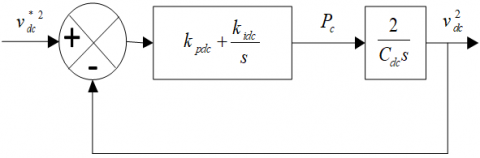Figure 10. DC voltage Regulation.

The closed loop transfer function is given in:

$G(s)=\frac{v_{dc}^{2}(s)}{v{{_{dc}^{*}}^{2}}(s)}=\frac{\frac{2{{k}_{pdc}}}{{{C}_{dc}}}s+\frac{2{{k}_{idc}}}{{{C}_{dc}}}}{{{s}^{2}}+\frac{2{{k}_{pdc}}}{{{C}_{dc}}}s+\frac{2{{k}_{idc}}}{{{C}_{dc}}}}$                  (58)

By the identification of (58) to a second order canonic transfer function (58):

$H(s)=\frac{2\zeta {{\omega }_{n}}s+\omega _{n}^{2}}{{{s}^{2}}+2\zeta {{\omega }_{n}}s+\omega _{n}^{2}}$             (59)

We can find:

\begin{align} & {{k}_{idc}}={{C}_{dc}}\omega _{n}^{2}/2 \\ & {{k}_{pdc}}=\xi {{\omega }_{n}}{{C}_{dc}} \\ \end{align}            (60)

$\omega_{n}=2 \pi f_{n}$ is the Natural pulsatance of the controller, and $0<\xi<1$ is the damping factor for a good dynamic and acceptable oscillations, we choose $f_{n}=25 \mathrm{~Hz} \xi=0.7$.

By the same method, we can also obtain the PI coefficients of currents, voltages and Boost controllers as summarized in Table 2 below.

Table 2. PI parameters

 Coefficients Value kpdc 22.7 Kidc 109 kpfp 3.5 Kifp 355 kpfs 0.3 Kifs 10 Kppv 0.45 Kipv 4.6
3. Results and Discussion

Harmonic current and voltage filtering, reactive power compensation and performance of the PVG-UPQC with the proposed control have been examined in Matlab/Simulink environment under nonlinear load variation and voltage sag/swell. The parameters used in the present study are shown in Table 3.

## 11a.png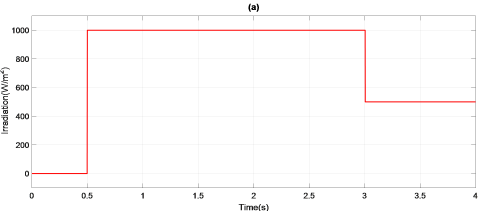## 11b.png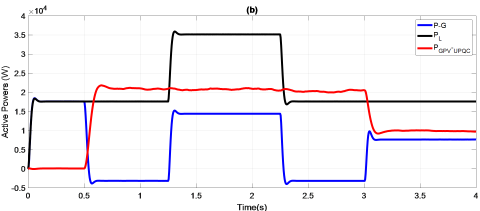## 11c.png## 11d.pngFigure 11. Simulation results under weather variation of the suggested controller. a): Variations of irradiations, b): Active power exchange of the PVG-UPQC under weather variations, c): Reactive power exchange of the PVG-UPQC under weather variations, d): DC-link voltage vdc

Table 3. System parameters

 Parameter value RMS value of the source voltage 220 V DC-link capacitor Cdc 8 mF Source impedance Rs, Ls 3 mΩ, 2.6 μH Shunt filter impedance Rfp, Lfp 20 mΩ, 2.5 mH Series filter impedance Rfs, Lfs,Cfs 1.5 Ω, 3 mH, 0.1 mF boost converter parameters Lpv,Cpv 5 mH, 55 mF Line impedance Rl, Ll 10 mΩ, 0.3 μH Diode rectifier load Rd , Ld 15 Ω, 2 mH PV array Ppv, Vmp,Imp, Isc,Voc 150W, 34.5V, 4.35A, 4.75A, 43.5V DC-link voltage reference 900 V Switching frequency fs 12 kHz kp1= kp2 1120 ks1= ks2 1150 Kb1= kb2 1450 kdc 250

Figure 11(a) illustrates the selected irradiation’s profile when the temperature is adjusted to 25°C. Initially, there is no irradiation (t<0.5s), the PVG-UPQC is controlled just to filtrate harmonics and compensate reactive power, while the load is fed by the grid (17.5 kW). Then, irradiation is adjusted to reach 1000 W/m2, in this case the injected maximum power is about 21 kW, in this case, the produced power is higher than the power required by the load, so the PVG-UPQC is controlled to filtrate harmonics, compensate reactive power, feed the load and inject power to the grid between [t =0.5s and t =1.25s] (see Figure 11(b) and (c)). From these figures, it is obvious that throughout the simulation, the consumed active and reactive powers (respectively 17.5 kW and 400 VAR) are equal to the sum of those delivered by the PVG-UPQC and the grid.

The load variation has a negligible effect on the DC-link voltage (about 2V), and it recovers in around 0.05s (see Figure 11(d)).

The dynamic behavior under an abrupt variation of load, by adding another load having the same value between [t=1.25s and t=2.25s] which is illustrated in Figures 12(a) and 12(b). It is clear to see that the grid current, after applying the control, is pure sinusoidal, moreover, even in this temporary state, the unity power factor goal is successfully reached.

## 12a.png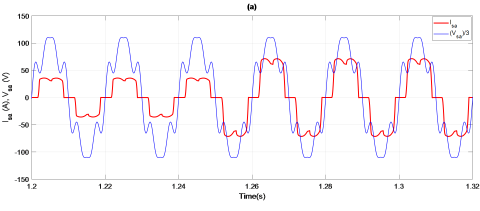## 12b.png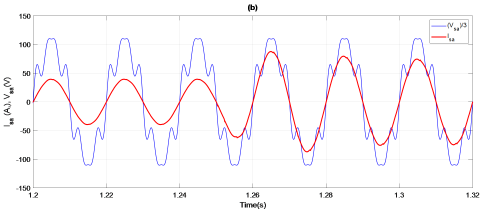## 12c.png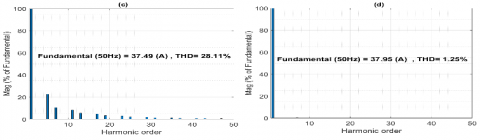## 12d.png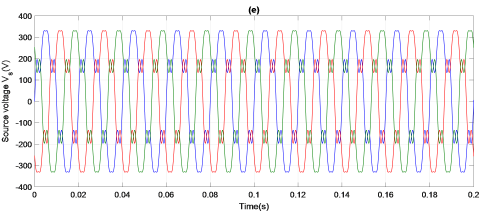## 12e.png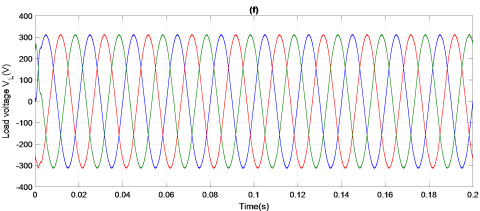## 12f.pngFigure 12. Simulation results under load variation of the suggested controller. a): A-phase source voltage with source current before compensation, b): A-phase source voltage with source current after compensation, c): Source current harmonic spectrum before compensation, d): Source current harmonic spectrum after compensation. e): Voltage of the load before compensation. f): Voltage of the load after compensation. g): Harmonic spectrum of load voltage before compensation, h): Load voltage harmonic spectrum after compensation

## 13a.png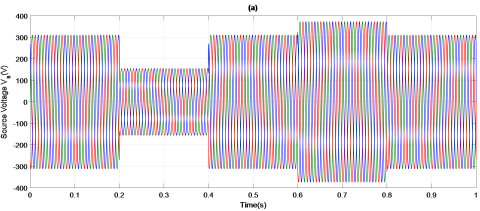## 13b.png## 13c.png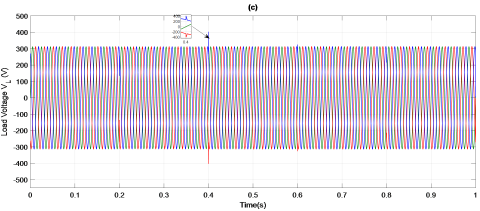Figure 13. Simulation results during a sag and a swell test of the suggested controller. a): Perturbed source voltage profile. b): Compensation voltage during perturbation. c): Voltage of the load after compensation

## 14a.png## 14b.png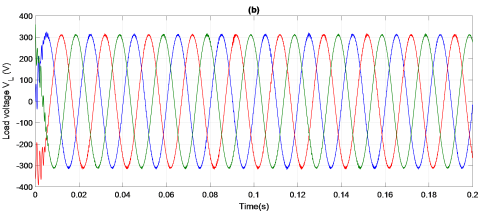## 14c.png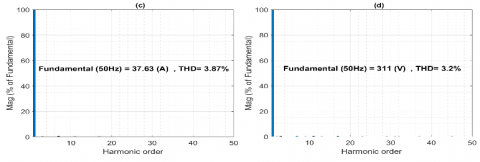## 14d.png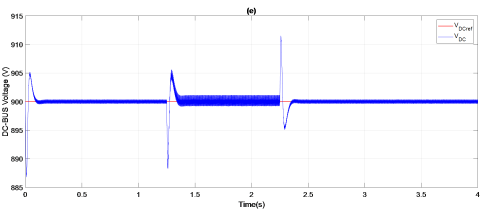Figure 14. Simulation results with PI controller. a): A-phase source voltage with source current after compensation, b): Voltage of the load after compensation. c): Source current harmonic spectrum after compensation, d): Load voltage harmonic spectrum after compensation. e): DC bus voltage vdc

From the sag and swell tests illustrated in Figures 13(a) and 13(b), it can be observed that the PVG-UPQC quickly injects equal positive voltage components in the case off sag which are phase-locked to the grid voltage, while in the case of voltage swell, the PVG-UPQC inject negative voltage components in opposite phase with the supply voltage to correct and maintain the load voltage close to its normal value (see Figure 13(c)).

In case of feedback control, the spectral analysis of AC grid current and the voltage of the load with and without compensation are presented in Figures 12(c) and (d), for current in Figures 12(g) and (h) for voltage. For linear control, the same spectrums are shown in Figures 14(c) and (d). It reveals that the UPQC decreases THD in the grid currents from 28.11% to 3.87% with the classic PI controller. whilst, with feedback linearisation based SVM controller, the current THD is decreased to 1.25%. The load voltage THD decreases from 24.59% to 3.2% with PI, while it is further decreased to 1.12% when feedback linearisation based SVM controller is applied which demonstrates the efficiency of the developed nonlinear controller.

The PVG-UPQC was tested with a sag and swell in the grid voltage, and the load voltage was again found quickly very close to a sinusoidal voltage. So, the PVG-UPQC is capable to deliver the required compensating voltage components to keep constant load voltage.

The absence of an overshoot in DC bus voltage response during load variation, rapidity and negligible THD, proves the superiority and the effectiveness of the feedback linearization based SVM controller compared to the traditional linear PI controller as presented in Table 4.

Table 4. Comparison of PI controller with FL-PDPC

 Factor PI controller FL-PDPC controller THDi (%) 3.87 1.25 THDv (%) 3.2 1.12 Charging of DC link (s) 0.11 0.05 Overshoot + -
4. Conclusion

In the present paper, a control design of the PVG-UPQC was carried out including its mathematical model and harmonic extraction methods for both currents and voltages. Feedback linearisation combined with predictive DPC controller is derived to suppress currents and voltages harmonics. For the close power switches control, a three-level SVM technique were used due to its benefits in terms of a fixed frequency of implementation. Simulation results divulge that in all stages of the system operation, the load side voltages and source side currents are very close to a sinusoidal shape. These results show clearly that PVG-UPQC controlled by feedback linearisation-based PDPC offers much better performance compared to the traditional controllers’ case.

Nomenclature
 Xfp X related to the parallel filter Xfs X related to the series filter P Active Power (W) Q Reactive Power (VAR) i Electrical Current (A) v Electrical Voltage (V) Abbreviation PVG Photovoltaic Generator UPQC Unified Power quality Conditioner SVM Space Vector Modulation DC Direct Current FL Feedback Linearisation THD Total Harmonic Distortion
References

 Gongati, P.R.R., Marala, R.R., Malupu, V.K. (2020). Mitigation of certain power quality issues in wind energy conversion system using UPQC and IUPQC devices. European Journal of Electrical Engineering, 22(6): 447-455. https://doi.org/10.18280/ejee.220606

 Kumar, P. (2020). Power quality investigation by reduced switching UPQC. European Journal of Electrical Engineering, 22(4-5): 335-347. https://doi.org/10.18280/ejee.224-505

 De Silva, H.J., Shafieipour, M. (2021). An improved passivity enforcement algorithm for transmission line models using passive filters. Electric Power Systems Research, 196: 107255. http://dx.doi.org/10.1016/j.epsr.2021.107255

 Patnaik, N., Panda, A.K. (2016). Performance analysis of a 3 phase 4 wire UPQC system based on PAC based SRF controller with real time digital simulation. International Journal of Electrical Power & Energy Systems, 74: 212-221. http://dx.doi.org/10.1016/j.ijepes.2015.07.027

 Gurrola-Corral, C., Segundo, J., Esparza, M., Cruz, R. (2020). Optimal LCL-filter design method for grid-connected renewable energy sources. International Journal of Electrical Power & Energy Systems, 120: 105998. http://dx.doi.org/10.1016/j.ijepes.2020.105998

 Hemalatha, R., Ramasamy, M. (2020). Microprocessor and PI controller based three phase CHBMLI based DSTATCOM for THD mitigation using hybrid control techniques. Microprocessors and Microsystems, 76: 103093. http://dx.doi.org/10.1016/j.micpro.2020.103093

 Bacon, V.D., da Silva, S.A.O., Guerrero, J.M. (2022). Multifunctional UPQC operating as an interface converter between hybrid AC-DC microgrids and utility grids. International Journal of Electrical Power & Energy Systems, 136: 107638. https://doi.org/10.1016/j.ijepes.2021.107638

 Dahdouh, A., Barkat, S., Chouder, A. (2016). A combined sliding mode space vector modulation control of the shunt active power filter using robust harmonic extraction method. Algerian Journal of Signals and Systems, 1(1): 37-46. https://doi.org/10.51485/ajss.v1i1.17

 Benaissa, A., Bouzidi, M., Barkat, S. (2012). Application of feedback linearization to the virtual flux direct power control of three-level three-phase shunt active power filter. International Review on Modelling and Simulations, 5(3): 1128-1140. https://ideas.repec.org/a/spr/ijsaem/v8y2017i2d10.1007_s13198-016-0433-3

 Koroglu, T., Tan, A., Savrun, M.M., Cuma, M.U., Bayindir, K.C., Tumay, M. (2019). Implementation of a novel hybrid UPQC topology endowed with an isolated bidirectional DC–DC converter at DC link. IEEE Journal of Emerging and Selected Topics in Power Electronics, 8(3): 2733-2746. http://dx.doi.org/10.1109/JESTPE.2019.2898369

 Yang, R.H., Jin, J.X. (2020). Unified power quality conditioner with advanced dual control for performance improvement of DFIG-based wind farm. IEEE Transactions on Sustainable Energy, 12(1): 116-126. https://doi.org/10.1109/TSTE.2020.2985161

 Devassy, S., Singh, B. (2020). Performance analysis of solar PV array and battery integrated unified power quality conditioner for microgrid systems. IEEE Transactions on Industrial Electronics, 68(5): 4027-4035. http://dx.doi.org/10.1109/TIE.2020.2984439

 Mansor, M.A., Hasan, K., Othman, M.M., Noor, S.Z.B. M., Musirin, I. (2020). Construction and performance investigation of three-phase solar PV and battery energy storage system integrated UPQC. IEEE Access, 8: 103511-103538. http://dx.doi.org/10.1109/ACCESS.2020.2997056

 Yu, J., Xu, Y., Li, Y., Liu, Q. (2020). An inductive hybrid UPQC for power quality management in premium-power-supply-required applications. IEEE Access, 8: 113342-113354. https://doi.org/10.1109/ACCESS.2020.2999355

 Prathyusha, D., Venkatesh, P. (2015). UVTG control strategy for three phase four wire UPQC to improve power quality. Int. Electr. Eng. J, 6(9): 1988-1993. https://doi.org/10.1016/j.ijepes.2022.108038

 da Silva, S.A.O., Campanhol, L.B.G., Pelz, G.M., de Souza, V. (2020). Comparative performance analysis involving a three-phase UPQC operating with conventional and dual/inverted power-line conditioning strategies. IEEE Transactions on Power Electronics, 35(11): 11652-11665. http://dx.doi.org/10.1109/TPEL.2020.2985322

 Poongothai, S., Srinath, S. (2020). Power quality enhancement in solar power with grid connected system using UPQC. Microprocessors and Microsystems, 79: 103300. http://dx.doi.org/10.1016/j.micpro.2020.103300

 Alam, S.J., Arya, S.R. (2020). Control of UPQC based on steady state linear Kalman filter for compensation of power quality problems. Chinese Journal of Electrical Engineering, 6(2): 52-65. https://doi.org/10.23919/CJEE.2020.000011

 Ozdemir, A., Ozdemir, Z. (2014). Digital current control of a three-phase four-leg voltage source inverter by using pqr theory. IET Power Electronics, 7(3): 527-539. http://dx.doi.org/10.1049/iet-pel.2013.0254

 Czarnecki, L.S. (2014). Constraints of instantaneous reactive power PQ theory. IET Power Electronics, 7(9): 2201-2208. http://dx.doi.org/10.1049/iet-pel.2013.0579

 Bharathi, S.L.K., Selvaperumal, S. (2020). MGWO-PI controller for enhanced power flow compensation using unified power quality conditioner in wind turbine squirrel cage induction generator. Microprocessors and Microsystems, 76: 103080. http://dx.doi.org/10.1016/j.micpro.2020.103080

 Dash, S.K., Ray, P.K. (2020). A new PV-open-UPQC configuration for voltage sensitive loads utilizing novel adaptive controllers. IEEE Transactions on Industrial Informatics, 17(1): 421-429. https://doi.org/10.1109/TII.2020.2986308

 Guzman, R., de Vicuna, L.G., Morales, J., Castilla, M., Matas, J. (2015). Sliding-mode control for a three-phase unity power factor rectifier operating at fixed switching frequency. IEEE Transactions on Power Electronics, 31(1): 758-769. http://dx.doi.org/10.1109/TPEL.2015.2403075

 Chebabhi, A., Fellah, M.K., Benkhoris, M.F., Kessal, A. (2015). Sliding mode controller for four leg shunt active power filter to eliminating zero sequence current, compensating harmonics and reactive power with fixed switching frequency. SJEE, 12(2): 205-218. http://dx.doi.org/10.2298/SJEE1502205C

 Ibrahim, Z.B., Hossain, M.L., Bugis, I.B., Lazi, J.M., Yaakop, N.M. (2014). Comparative analysis of PWM techniques for three level diode clamped voltage source inverter. International Journal of Power Electronics and Drive Systems, 5(1): 15-23. http://dx.doi.org/10.11591/ijpeds.v5i1.6038

 Han, J., Li, X., Jiang, Y., Gong, S. (2020). Three-phase UPQC topology based on quadruple-active-bridge. IEEE Access, 9: 4049-4058. https://doi.org/10.1109/ACCESS.2020.3047961

 Reisi, A.R., Moradi, M.H., Showkati, H. (2013). Combined photovoltaic and unified power quality controller to improve power quality. Solar Energy, 88: 154-162. https://doi.org/10.1016/j.solener.2012.11.024